# Inductor

Inductor is an electrical component that stores energy in magnetic field.

The inductor is made of a coil of conducting wire.

In an electrical circuit schematics, the inductor marked with the letter L.

The inductance is measured in units of Henry [L].

Inductor reduce current in AC circuits and short circuit in DC circuits.

### Inductor picture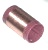### Inductor symbols

##### Inductor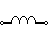##### Iron core inductor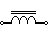##### Variable inductor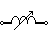### Inductors in series

For several inductors in series the total equivalent inductance is:

LTotal = L1+L2+L3+...

### Inductors in parallel

For several inductors in parallel the total equivalent inductance is: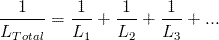### Inductor's voltage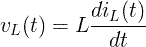### Inductor's current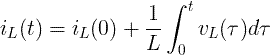### Energy of inductor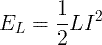### AC circuits

XL = ωL

##### Inductor's impedance

Cartesian form:

ZL = jXL = jωL

Polar form:

ZL = XL∠90º

An inductor is a passive two-terminal electrical component that stores energy in a magnetic field. When the current through the inductor changes, the magnetic field does too, inducing a voltage across the terminals. The inductor is often used in electrical circuits to delay or prevent changes in current flow.

Inductors are made from a coil of wire wrapped around a magnetic core. The core can be made from iron, nickel, or any other magnetic material. The amount of inductance depends on the number of turns of wire, the diameter of the wire, and the type of core material.

Inductors are used in a variety of electrical circuits, including power supplies, transformers, and filters. In power supplies, inductors are used to smooth out the current flow and prevent voltage spikes. In transformers, inductors are used to step up or step down the voltage. In filters, inductors are used to remove noise and interference from signals.